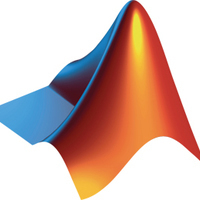# MATLAB

A high-level language and interactive environment for numerical computation, visualization, and programming
729
619
+ 1
34

## What is MATLAB?

Using MATLAB, you can analyze data, develop algorithms, and create models and applications. The language, tools, and built-in math functions enable you to explore multiple approaches and reach a solution faster than with spreadsheets or traditional programming languages, such as C/C++ or Java.
MATLAB is a tool in the Languages category of a tech stack.

## Who uses MATLAB?

Pros of MATLAB
18
5
Functions, statements, plots, directory navigation easy
4
Model based software development
3
S-Functions
2
REPL
1
Simple variabel control
1
Solve invertible matrix

## MATLAB Alternatives & Comparisons

What are some alternatives to MATLAB?
R Language
R provides a wide variety of statistical (linear and nonlinear modelling, classical statistical tests, time-series analysis, classification, clustering, ...) and graphical techniques, and is highly extensible.
NumPy
Besides its obvious scientific uses, NumPy can also be used as an efficient multi-dimensional container of generic data. Arbitrary data-types can be defined. This allows NumPy to seamlessly and speedily integrate with a wide variety of databases.
Octave
It is software featuring a high-level programming language, primarily intended for numerical computations. Octave helps in solving linear and nonlinear problems numerically, and for performing other numerical experiments using a language that is mostly compatible with MATLAB.
Tableau
Tableau can help anyone see and understand their data. Connect to almost any database, drag and drop to create visualizations, and share with a click.
Python
Python is a general purpose programming language created by Guido Van Rossum. Python is most praised for its elegant syntax and readable code, if you are just beginning your programming career python suits you best.
See all alternatives
MATLAB's Followers
619 developers follow MATLAB to keep up with related blogs and decisions.